×#### Thank you for registering.

One of our academic counsellors will contact you within 1 working day.

Click to Chat

1800-1023-196

+91-120-4616500

CART 0

• 0

MY CART (5)

Use Coupon: CART20 and get 20% off on all online Study Material

ITEM
DETAILS
MRP
DISCOUNT
FINAL PRICE
Total Price: Rs.

There are no items in this cart.
Continue Shopping• Complete Physics Course - Class 11
• OFFERED PRICE: Rs. 2,968
• View Details

```IIT JEE Ray Optics Study Material

Table of Content

Light Rays and Beams

Reflection of Light

Laws of Reflection

Deviation of Light by Plane Mirror

Deviation  of  light  due  to rotation of a mirror

Image in a plane mirror

Virtual and Real Images

Characteristics of the Image Formed by a Plane Mirror

Refraction

Problem (JEE Main)

Related Resources

Light Rays and Beams

A ray of light is the direction along which the light energy travels. In practice a ray has a finite width and is represented in diagrams as straight lines. A beam of light is a collection of rays. A search light emits a parallel beam of light (Fig.a). Light from a lamp travels in all directions which is a divergent beam. (Fig.b). A convex lens produces a convergent beam of light, when a parallel beam falls on it (Fig.c).Reflection of Light

Highly polished metal surfaces reflect about 80% to 90% of the light incident on them. Mirrors in everyday use are therefore usually made of depositing silver on the backside of the glass. The largest reflector in the world is a curved mirror nearly 5 metres across, whose front surface is coated with aluminium. It is the hale Telescope on the top of Mount Palomar, California, U.S.A. Glass by itself, will also reflect light, but the percentage is small when compared with the case of silvered surface. It is about 5% for an air-glass surface.Laws of Reflection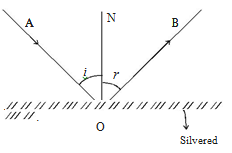Consider a ray of light, AO, incident on a plane mirror XY at O. It is reflected along OB. Let the normal ON is drawn at the point of incidence. The angle AON between the incident ray and the normal is called angle of incidence,i (as shown in the figure) is the angle BON between the reflected ray and the normal is called angle of reflection, r. Experiments show that :

(i) The incident ray, the reflected ray and the normal drawn to the reflecting surface at the point of incidence, all lie in the same plane.

(ii) The angle of incidence is equal to the angle of reflection. (i.e) i = r.

These are called the laws of reflection.

Refer this video to know more about laws of reflection

Deviation of Light by Plane Mirror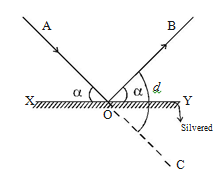Consider a ray of light, AO, incident on a plane mirror XY (side figure) at O. It is reflected along OB. The angle AOX made by AO with XY is known as the glancing angle α with the mirror. Since the angle of reflection is equal to the angle of incidence, the glancing angle BOY made by the reflected ray OB with the mirror is also equal to α.

The light has been deviated from a direction AO to a direction OB. Since angle COY = angle AOX, it follows that angle of  deviation, d = 2α.

So, in general, the angle of deviation of a ray by a plane mirror or a plane surface is twice the glancing angle.

Deviation  of  light  due  to rotation of a mirror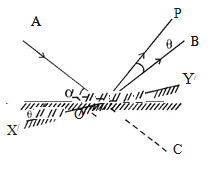Let us consider a ray of light AO incident on a plane mirror XY at O. It is reflected along OB. Let α be the glancing angle with XY (as shown in figure). We know that the angle of deviation COB = 2α.

Suppose the mirror is rotated through an angle θ to a position X′Y′. The same incident ray AO is now reflected along OP. Here the glancing angle with X′Y′ is (α + θ). Hence the new angle of deviation COP = 2 (α + θ). The reflected ray has thus been rotated through an angle BOP when the mirror is rotated through an angle θ.

∠BOP = ∠COP – ∠COB

∠BOP = 2 (α + θ) – 2α = 2θ

For the same incident ray, when the mirror is rotated through an angle, the reflected ray is rotated through twice the angle.

Image in a plane mirror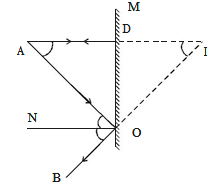Let us consider a point object A placed in front of a plane mirror M as shown in the figure. Consider a ray of light AO from the point object incident on the mirror and reflected along OB. Draw the normal ON to the mirror at O.

The angle of incidence AON = angle of reflection BON

Another ray AD incident normally on the mirror at D is reflected back along DA. When BO and AD are produced backwards, they meet at I. Thus the rays reflected from M appear to come from a point I behind the mirror.

From the figure,

∠AON = ∠DAO, alternate angles and ∠BON = ∠DIO, corresponding angles it follows that ∠DAO = ∠DIO

The triangles ODA and ODI are congruent ∴ AD = ID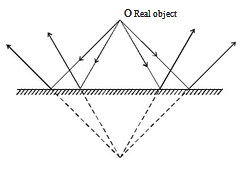For a given position of the object, A and D are fixed points. Since AD = ID, the point I is also fixed. It should be noted that AO = OI. So the object and its image in a plane mirror are at equal perpendicular distances from the mirror.

Virtual and Real Images

An object placed in front of a plane mirror has an image behind the mirror. The rays reflected from the mirror do not actually meet through I, but only appear to meet M and the image cannot be received on the screen, because the image cannot be received on the screen, because the image is behind the mirror. This type of image is called an unreal or virtual image as shown in figure.If a convergent beam is incident on a plane mirror, the reflected rays pass through a point I in front of M, as shown in the figure. In the figure a real object (divergent beam) gives rise to a virtual image. In the figure, a virtual object (convergent beam) gives a real image. Hence plane mirrors not only produce virtual images for real objects but also produce real images for virtual objects.

Characteristics of the Image Formed by a Plane Mirror

(a) Image formed by a plane mirror is as far behind the mirror as the object is in front of it and it is always virtual.

(b) The image produced is laterally inverted.

(c) The minimum size of the mirror required to see the complete image of the object is half the size of the object.

(d) If the mirror turns by an angle θ, the reflected ray turns through an angle 2θ.

(e) If an object is placed between two plane mirrors inclined at an angle θ, then the number of images formed is n = (360º/θ) – 1

Refraction:The concept of refraction and the index of refraction are of immense importance. If light travels from point A to point B, then its speed will be highest if it travels in a straight line. But it has to pass through various materials then it will pass through them at different speeds and the motion will not be in a straight line. Hence, when it enters a new medium, it gets bent a bit and this bending is termed as refraction. The human eye always assumes light to be traveling in a straight line and hence when an object appears to be bent slightly due to refraction, we assume that the object is bent and not the light.The index of refraction depends on the medium through which light passes. The speed of light is more in medium which are less optically dense and less in more optically dense mediums. The mathematical formula for calculation of index of refraction is:

n = Speed of light in vacuum/ Speed of light in the medium = c/v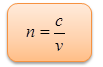The index of refraction is represented by the letter ‘n’ and denotes the angle at which the light bends. When light travels from a medium with refractive index n1 to the other with refractive index n2, the relation between the angle of incidence θ1 and the angle of refraction θ2 is given by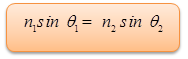Since the speed of light in air is almost the same as the speed of light in a vacuum, so in most of the cases a value of one for the index of refraction of air. We have listed the indices of refraction for various substances in the table given below:

Substance (at 20° C)

Index of refraction, n

Diamond

2.419

Sodium Chloride

1.544

Water

1.333

Benzene

1.501The angle of incidence and angle of reflection is equal.

The incident ray, reflected ray and normal to the point of reflection lie in the same plane.

The law of reflection is applicable to all types of reflecting surface.

A plane mirror always forms virtual and erect image.

The distance of image and that of object is equal from the mirror.

The image formed by a plane mirror is laterally inverted.

Light traveling from a more dense to a less dense medium refract away from the normal. This behavior is exhibited by light moving from water to air.

Light traveling from a less dense to a more dense medium refract towards the normal. This behavior is exhibited by light moving from air into water.

The greater the difference in density* between the two media, the greater the refraction.

The greater the incident angle, the greater the refraction.

For light traveling from a more dense to a less dense medium, there is a critical angle* beyond which the light will not pass through the interface. A light ray with an angle of incidence equal to or greater than the critical angle will reflect, following the law of reflection.

Problem (JEE Main):

When an object is at distance x and y from a lens, a real image and a virtual image is formed respectively having some magnification. The focal length of the lens is

(a) (x+y)/2        (b) x – y

(c) √xy           (d) x + y

Solution:

The given lens is a convex lens. Let the magnification be m, then for real image

(1/mx) + (1/x) = 1/f        …... (1)

and for virtual image (1/-my) + (1/y) = 1/f      …...(2)

From Eq.(1) and Eq.(2), we get,

f = (x + y) / 2

From the above observation, we conclude that, option (a) is correct.Question 1

Light is a form of energy produced by a ______.

(a) luminous object

(b) transparent object

(c) non-luminous object

(d) opaque object

Question 2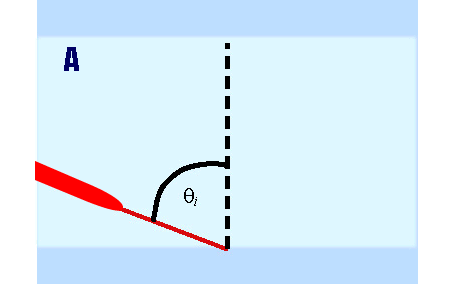The phenomenon by which the incident light falling on a surface is sent back into the same medium is known as ________.

(a) polarization

(b) reflection

(c) refraction

(d) absorption

Question 3

An object becomes invisible when it undergoes ______ reflection.

(a) regular

(b) irregular

(c) diffused

(d) normal

Question 4

The centre of the sphere of which the spherical mirror forms a part is called ____________.

(a) centre of curvature

(b) focus

(c) pole

(d) vortex

Question 5

The image formed by a plane mirror is always _______.

(a) real and erect

(b) virtual and erect

(c) real and inverted

(d) virtual and invertedQ.1
Q.2
Q.3
Q.4
Q.5

a

b

a

a

b

Related Resources:-

You might like to magnification and thin lens.

For getting an idea of the type of questions asked, refer the  Previous Year Question Papers.

To read more, Buy study materials of Ray Optics and Optical Instruments comprising study notes, revision notes, video lectures, previous year solved questions etc. Also browse for more study materials on Physics here.
```### Course Features

• 101 Video Lectures
• Revision Notes
• Previous Year Papers
• Mind Map
• Study Planner
• NCERT Solutions
• Discussion Forum
• Test paper with Video Solution